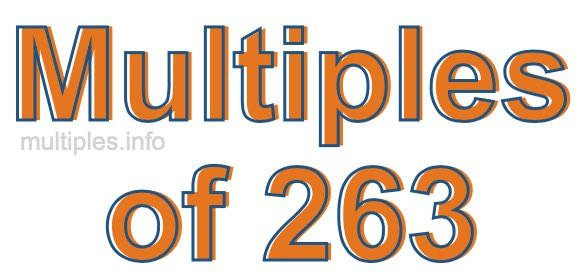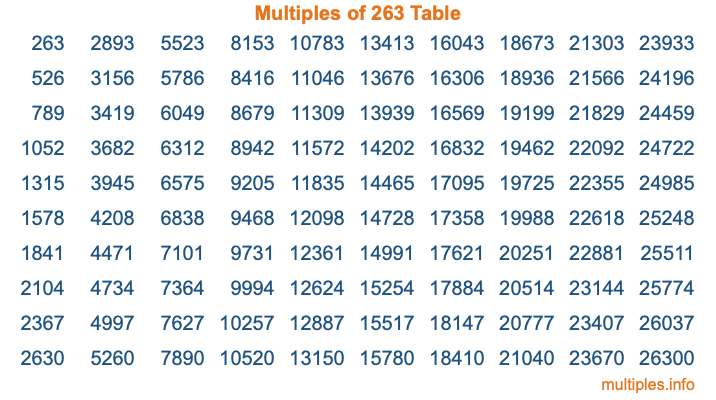Multiples of 263Welcome to the Multiples of 263 page. Here we will first teach you everything you will ever need to know about the multiples of 263, and then give you a study guide summary of everything we taught you to make sure you remember it all. Use this page to look up facts and learn information about the multiples of 263. This page will make you a multiples of two hundred sixty-three expert!

Definition of Multiples of 263
Multiples of 263 are all the numbers that when divided by 263 equal an integer. Each of the multiples of 263 are called a multiple. A multiple of 263 is created by multiplying 263 by an integer.

Therefore, to create a list of multiples of 263, you start with 1 multiplied by 263, then 2 multiplied by 263, then 3 multiplied by 263, and so on for as long as you want. Thus, the list of the first five multiples of 263 is 263, 526, 789, 1052, and 1315. To see a larger list of multiples of 263, see the printable image of Multiples of 263 further down on this page. We also have a category where you can choose any nth multiple of 263.

Multiples of 263 Checker
The Multiples of 263 Checker below checks to see if any number of your choice is a multiple of 263. In other words, it checks to see if there is any number (integer) that when multiplied by 263 will equal your number. To do that, we divide your number by 263. If the the quotient is an integer, then your number is a multiple of 263.

Is  a multiple of 263?

Least Common Multiple of 263 and ...
A Least Common Multiple (LCM) is the lowest multiple that two or more numbers have in common. This is also called the smallest common multiple or lowest common multiple and is useful to know when you are adding our subtracting fractions. Enter one or more numbers below (263 is already entered) to find the LCM.

Check out our LCM Calculator if you need more details about the Least Common Multiple or if you need the LCM for different numbers for adding and subtraction fractions.

nth Multiple of 263
As we stated above, 263 is the first multiple of 263, 526 is the second multiple of 263, 789 is the third multiple of 263, and so on. Enter a number below to find the nth multiple of 263.

th multiple of 263

Multiples of 263 vs Factors of 263
263 is a multiple of 263 and a factor of 263, but that is where the similarities end. All postive multiples of 263 are 263 or greater than 263. All positive factors of 263 are 263 or less than 263.

Below is the beginning list of multiples of 263 and the factors of 263 so you can compare:

Multiples of 263: 263, 526, 789, 1052, 1315, etc.

Factors of 263: 1, 263

As you can see, the multiples of 263 are all the numbers that you can divide by 263 to get a whole number. The factors of 263, on the other hand, are all the whole numbers that you can multiply by another whole number to get 263.

It's also interesting to note that if a number (x) is a factor of 263, then 263 will also be a multiple of that number (x).

Multiples of 263 vs Divisors of 263
The divisors of 263 are all the integers that 263 can be divided by evenly. Below is a list of the divisors of 263.

Divisors of 263: 1, 263

The interesting thing to note here is that if you take any multiple of 263 and divide it by a divisor of 263, you will see that the quotient is an integer.

Multiples of 263 Table
Below is an image of the first 100 multiples of 263 in a table. The table is in chronological order, column by column. The first column has the first ten multiples of 263, the second column has the next ten multiples of 263, and so on.The Multiples of 263 Table is also referred to as the 263 Times Table or Times Table of 263. You are welcome to print out our table for your studies.

Negative Multiples of 263
Although not often discussed or needed in math, it is worth mentioning that you can make a list of negative multiples of 263 by multiplying 263 by -1, then by -2, then by -3, and so on, to get the following list of negative multiples of 263:

-263, -526, -789, -1052, -1315, etc.

Multiples of 263 Summary
Below is a summary of important Multiples of 263 facts that we have discussed on this page. To retain the knowledge on this page, we recommend that you read through the summary and explain to yourself or a study partner why they hold true.

There are an infinite number of multiples of 263.

A multiple of 263 divided by 263 will equal a whole number.

263 divided by a factor of 263 equals a divisor of 263.

The nth multiple of 263 is n times 263.

The largest factor of 263 is equal to the first positive multiple of 263.

263 is a multiple of every factor of 263.

263 is a multiple of 263.

A multiple of 263 divided by a divisor of 263 equals an integer.

263 divided by a divisor of 263 equals a factor of 263.

Any integer times 263 will equal a multiple of 263.

Multiples of a Number
Here you can get the multiples of another number, all with the same attention to detail as we did for multiples of 263 on this page.

Multiples of
Multiples of 264
Did you find our page about multiples of two hundred sixty-three educational? Do you want more knowledge? Check out the multiples of the next number on our list!